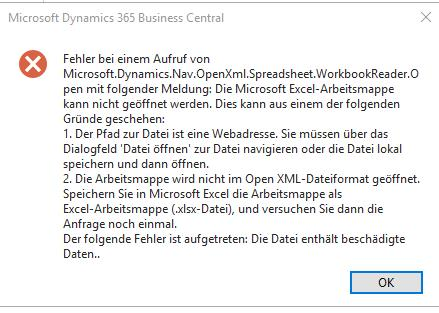# XLSX file can't be read by Microsoft OpenXML

We have implemented an interface using GemBox Spreadsheet Version 45.0.35.1131.
The interface saves the data to an XLSX file, using the following code:

``````Workbook.Save("example.xlsx");
``````

On the other end of the interface our client gets the following error (in German):The current workaround is to open the XLSX file in Microsoft Excel, saving it as an XLS file and then saving it as an XLSX file again.

Should we be using a different way to save the XLSX file?

Hi MKruck,

I’m afraid I was unable to reproduce this.

``````static void Main()
{
var path = "example.xlsx";

// Write Excel file with GemBox.Spreadsheet.
var workbook = new ExcelFile();

for (int r = 0; r < 50; r++)
for (int c = 0; c < 10; c++)
worksheet.Cells[r, c].Value = c + r * 10;

workbook.Save(path);

// Read Excel file with OpenXML SDK.
.Worksheet.Elements<SheetData>().First()
.Elements<Row>();

foreach (var row in rows)
{
foreach (var cell in row.Elements<Cell>())
Console.Write(\$"{cell.CellValue.Text,3}  ");
Console.WriteLine();
}
}
``````